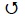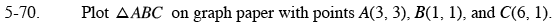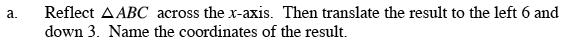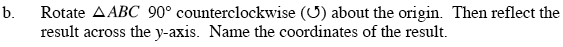### Home > GC > Chapter 5 > Lesson 5.3.1 > Problem5-70

5-70.
1. Plot ΔABC on graph paper with points A(3, 3), B(1, 1), and C(6, 1). 5-70 HW eTool (Desmos). Homework Help ✎

1. Reflect ΔABC across the x-axis. Then translate the result to the left 6 and down 3. Name the coordinates of the result.

2. Rotate ΔABC 90° counterclockwise () about the origin. Then reflect the result across the y-axis. Name the coordinates of the result.Reference Math Notes boxes in Lessons 1.2.2 and 1.2.3 if you need more help with reflection.

A''(3,3), B''(1,1), C''(1,6)

Use the eTool below to solve the problem.
Click the link at right for the full version of the eTool: GC 5-70 HW eTool